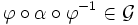# Normal fusion subsystem

A fusion subsystem$\mathcal{G}$ of a fusion system$\mathcal{F}$ on a group of prime power order$P$ is termed a normal fusion subsystem if:
• The subgroup$Q$ of$P$ for which$\mathcal{G}$ is a fusion system is a strongly closed subgroup of$P$. In other words, for any$\varphi:R \to P$ with$\varphi \in \mathcal{F}$,$\varphi(Q \cap R) \le Q$.
• Conjugation of any morphism in$\mathcal{G}$ by a morphism in$\mathcal{F}$ gives a morphism in$\mathcal{G}$, in the following sense: If$\varphi \in \mathcal{F}$ and$\alpha \in \mathcal{G}$ are morphisms such that$\varphi \circ \alpha \circ \varphi^{-1}$ is well-defined and between two objects of$\mathcal{G}$ (i.e., two subgroups of$Q$), then$\varphi\circ \alpha \circ \varphi^{-1} \in \mathcal{G}$.